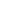# Represent equal groups with tape diagrams, and relate to repeated addition

EngageNY 60 min(s)
In this lesson, students understand that numbers other than 1, 10, and 100 can serve as units, and students represent the total of a given number of units with tape diagrams or using repeated addition, e.g., 2 + 2 + 2 + 2 = 8.